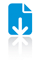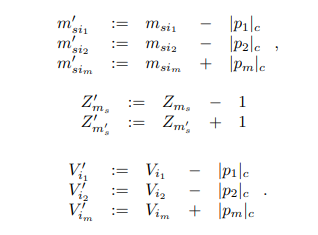# Tight Bounds for Online Graph Partitioning

ContentAbstract

We consider the following online optimization problem. We are given a graph G and each vertex of the graph is assigned to one of ` servers, where servers have capacity k and we assume that the graph has ` · k vertices. Initially, G does not contain any edges and then the edges of G are revealed one-by-one. The goal is to design an online algorithm ONL, which always places the connected components induced by the revealed edges on the same server and never exceeds the server capacities by more than �k for constant � > 0. Whenever ONL learns about a new edge, the algorithm is allowed to move vertices from one server to another. Its objective is to minimize the number of vertex moves. More specifically, ONL should minimize the competitive ratio: the total cost ONL incurs compared to an optimal offline algorithm OPT. The problem was recently introduced by Henzinger et al. (SIGMETRICS’2019) and is related to classic online problems such as online paging and scheduling. It finds applications in the context of resource allocation in the cloud and for optimizing distributed data structures such as union– find data structures. Our main contribution is a polynomial-time randomized algorithm, that is asymptotically optimal: we derive an upper bound of O(log ` + log k) on its competitive ratio and show that no randomized online algorithm can achieve a competitive ratio of less than Ω(log ` + log k). We also settle the open problem of the achievable competitive ratio by deterministic online algorithms, by deriving a competitive ratio of Θ(` log k); to this end, we present an improved lower bound as well as a deterministic polynomial-time online algorithm. Our algorithms rely on a novel technique which combines efficient integer programming with a combinatorial approach for maintaining ILP solutions. More precisely, we use an ILP to assign the connected components induced by the revealed edges to the servers; this is similar to existing approximation schemes for scheduling algorithms. However, we cannot obtain our competitive ratios if we run the ILPTop
Authors
• Henzinger, Monika
• Neumann, Stefan
• Räcke, Harald
• Schmid, StefanTop
Supplemental Material
Shortfacts
 Category Paper in Conference Proceedings or in Workshop Proceedings (Paper) Event Title ACM-SIAM Symposium on Discrete Algorithms (SODA) Divisions Subjects Event Location Alexandria, Virgina, USA Event Type Conference Event Dates January 2021 Date January 2021 Export BibTeX ASCII Citation DIDL Dublin Core EP3 XML EP3 XML with files embedded EndNote Eprints Application Profile HTML Citation JSON METS MODS Multiline CSV OAI-ORE Resource Map (Atom Format) OAI-ORE Resource Map (RDF Format) OpenURL ContextObject RDF+N-Triples RDF+N3 RDF+XML Refer Reference Manager Simple MetadataTop